# The More Power At Stake, The Stronger Instrumental Convergence Gets For Optimal Policies

8 min read11th Jul 20211 comment

# 29

Instrumental ConvergenceAI
Frontpage

Environmental Structure Can Cause Instrumental Convergence explains how power-seeking incentives can arise because there are simply many more ways for power-seeking to be optimal, than for it not to be optimal. Colloquially, there are lots of ways for "get money and take over the world" to be part of an optimal policy, but relatively few ways for "die immediately" to be optimal. (And here, each "way something can be optimal" is a reward function which makes that thing optimal.)

But how strong is this effect, quantitatively?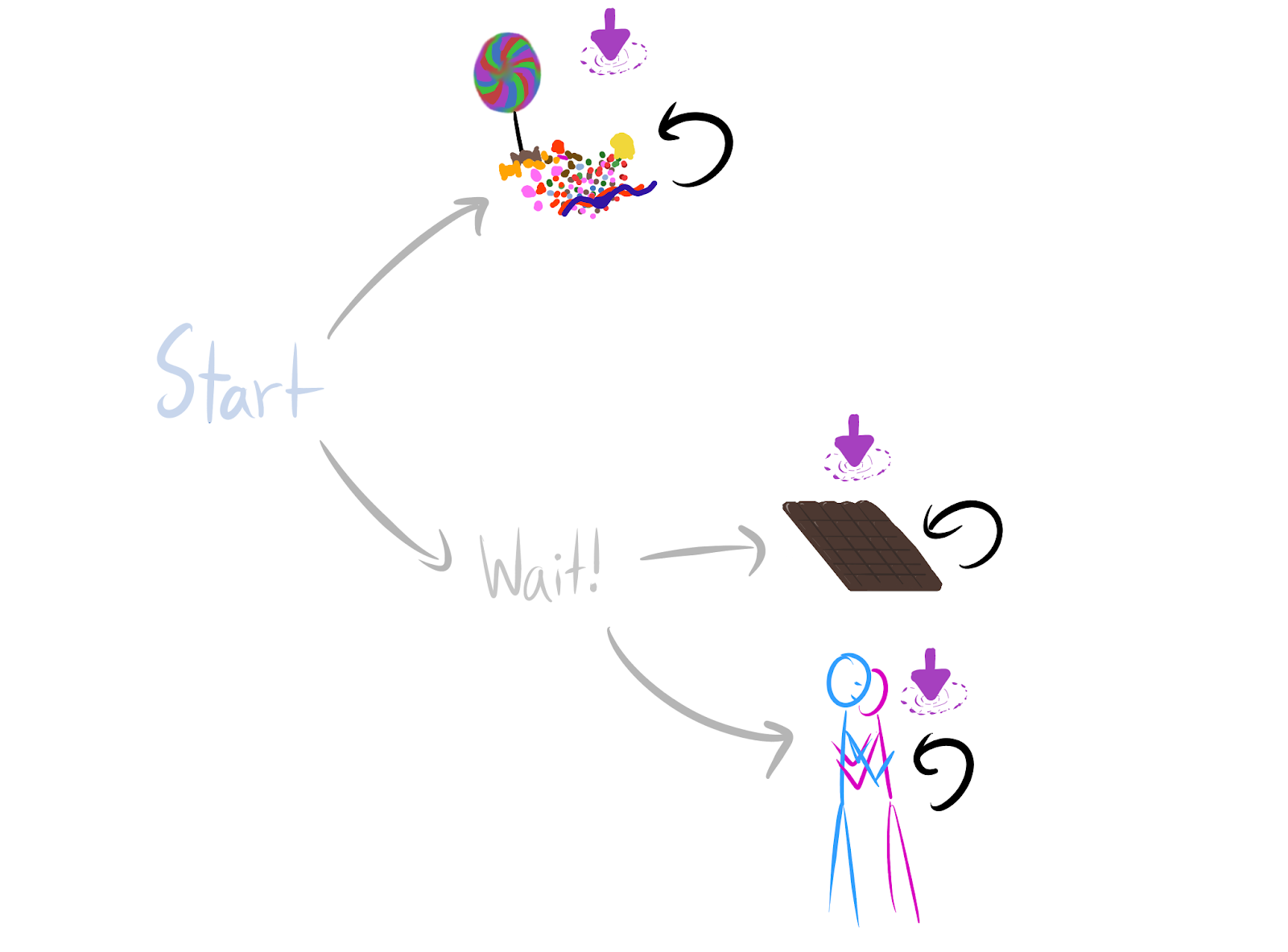Intuitively, it seems like there are twice as many ways for Wait! to be optimal (in the undiscounted setting, where we don't care about intermediate states).

In Environmental Structure Can Cause Instrumental Convergence, I speculated that we should be able to get quantitative lower bounds on how many objectives incentivize power-seeking actions:

Definition. At state , most reward functions incentivize action  over action  when for all reward functions , at least half of the orbit agrees that  has at least as much action value as  does at state .

...

What does 'most reward functions' mean quantitatively - is it just at least half of each orbit? Or, are there situations where we can guarantee that at least three-quarters of each orbit incentivizes power-seeking? I think we should be able to prove that as the environment gets more complex, there are combinatorially more permutations which enforce these similarities, and so the orbits should skew harder and harder towards power-incentivization.

Scaling law for instrumental convergence (informal): if policy set  lets you do " times as many things" than policy set  lets you do, then for every reward function, A is optimal over B for at least  of its permuted variants (i.e. orbit elements)

For example,  might contain the policies where you stay alive, and  may be the other policies: the set of policies where you enter one of several death states.

(Conjecture which I think I see how to prove: for almost all reward functions, A is strictly optimal over B for at least  of its permuted variants.)At least 22+1=23 of the orbit of every reward function agrees that Wait! is optimal (for average per-timestep reward). That's because there are twice as many ways for Wait! to be optimal over candy, than for the reverse to be true.

Basically, when you could apply the previous results, but "multiple times", you can get lower bounds on how often the larger set of things is optimal: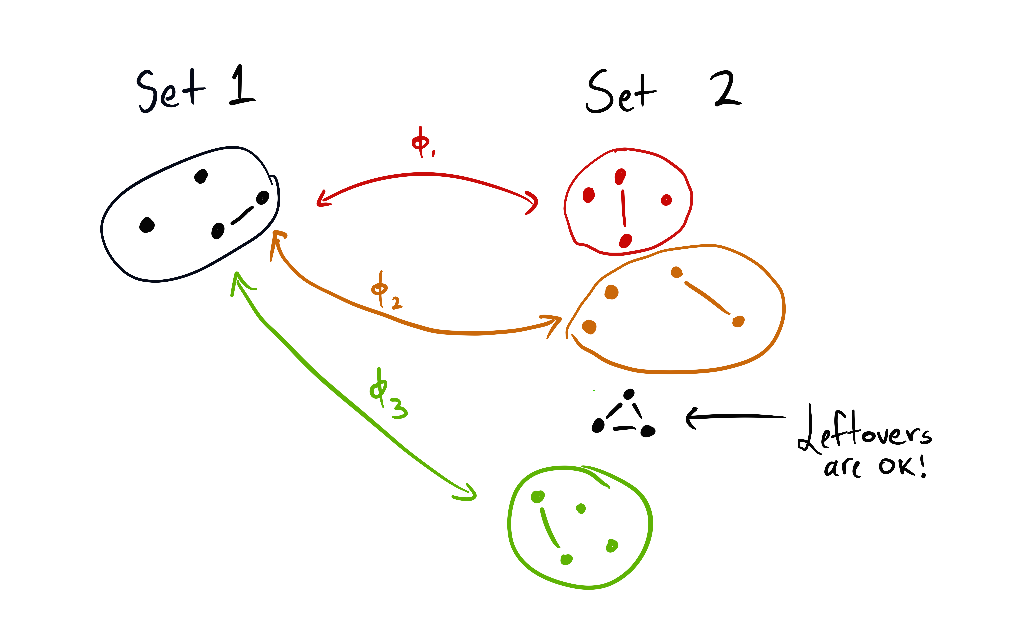Each set contains a subset of the agent's "options." A vertex is just the agent staying at that state. A linked pair is the agent alternating back and forth between two states. The triangle is a circuit of three states, which the agent can navigate as they please. Roughly, the theorem says: if the set 1 of options can be embedded 3 times into another set 2 of options (where the images are disjoint), then at least 33+1=34 of all variations on all reward functions agree that set 2 is optimal.

And in way larger environments - like the real world, where there are trillions and trillions of things you can do if you stay alive, and not much you can do otherwise - nearly all orbit elements will make survival optimal.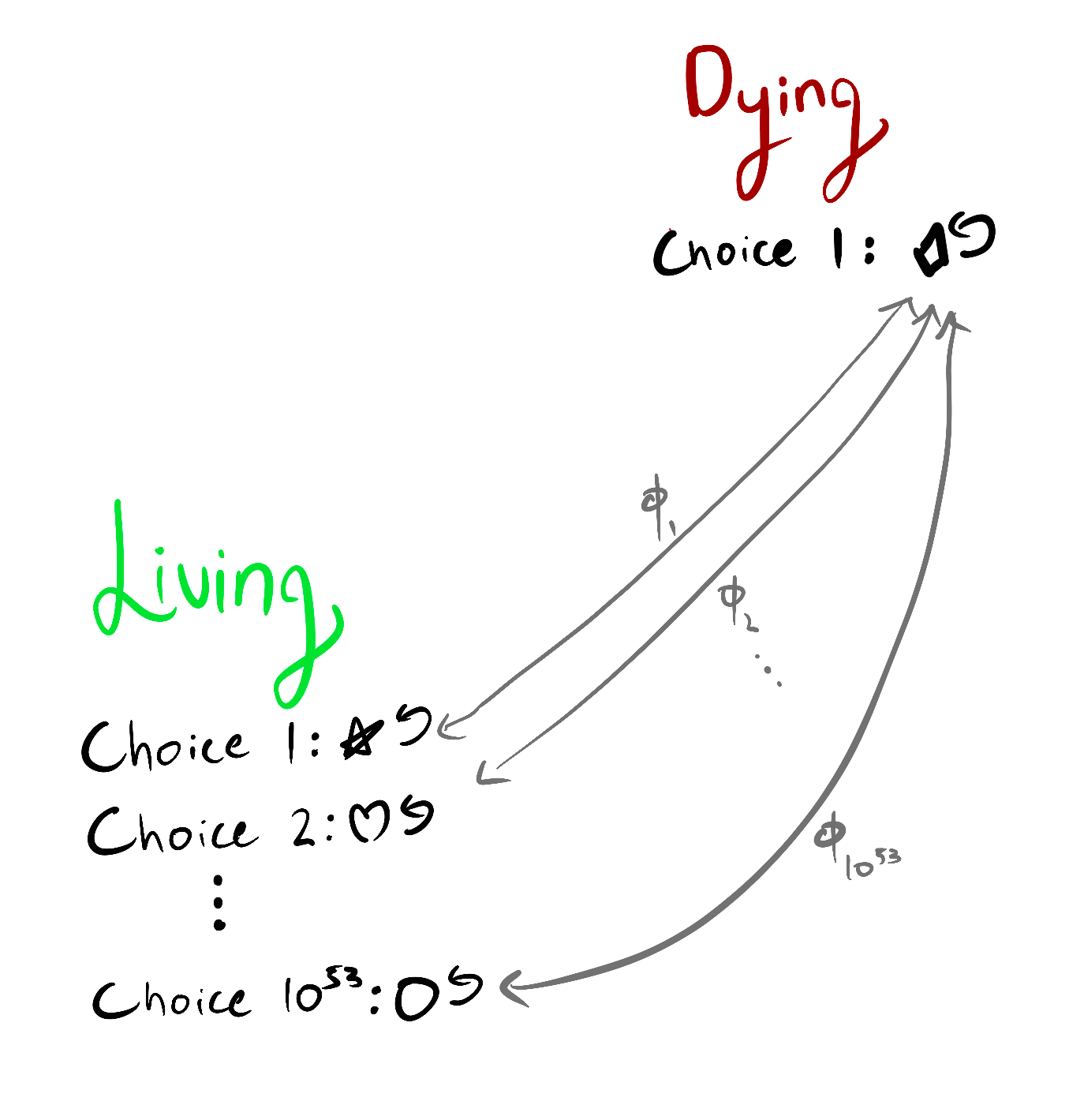In this environment, it's (average-reward) optimal to stay alive for at least 10531053+1 of the variants on each objective function.

I see this theory as beginning to link the richness of the agent's environment, with the difficulty of aligning that agent: for optimal policies, instrumental convergence strengthens proportionally to the ratio of .

# Why this is true

Optional section.

The proofs are currently in an Overleaf; let me know if you want access. But here's one intuition, using the candy/chocolate/reward example environment.

• Consider any reward function which says candy is strictly optimal.
• Then candy is strictly optimal over both chocolate and hug.
• We have two permutations: one switching the reward for candy and chocolate, and one switching reward for candy and hug.
• Each permutation produces a different orbit element (a different reward function variant).
• The permuted variants both agree that Wait! is strictly optimal.
• So there are at least twice as many orbit elements for which Wait! is strictly optimal over candy, than those for which candy is strictly optimal over Wait!.
• Either one of Start's child states (candy/Wait!) is strictly optimal, or they're both optimal. If they're both optimal, Wait! is optimal. Otherwise, Wait! makes up at least  of the orbit elements for which strict optimality holds.

## Conjecture

Fractional scaling law for instrumental convergence (informal): if staying alive lets you do  "things" and dying lets you do  "things", then for every reward function, staying alive is optimal for at least  of its orbit elements

I'm reasonably confident this is true, but I haven't worked through the combinatorics yet. This would slightly strengthen the existing lower bounds in certain situations. For example, suppose dying gives you 2 choices of terminal state, but living gives you 51 choices. The current result only lets you prove that at least  of the orbit incentivizes survival. The fractional lower bound would slightly improve this to .

# Invariances

In certain ways, the results are indifferent to e.g. increased precision in agent sensors: it doesn't matter if dying gives you 1 option and living gives you  options, or if dying gives you 2 options and living gives you  options.Wait! has twice as many ways of being average-optimal.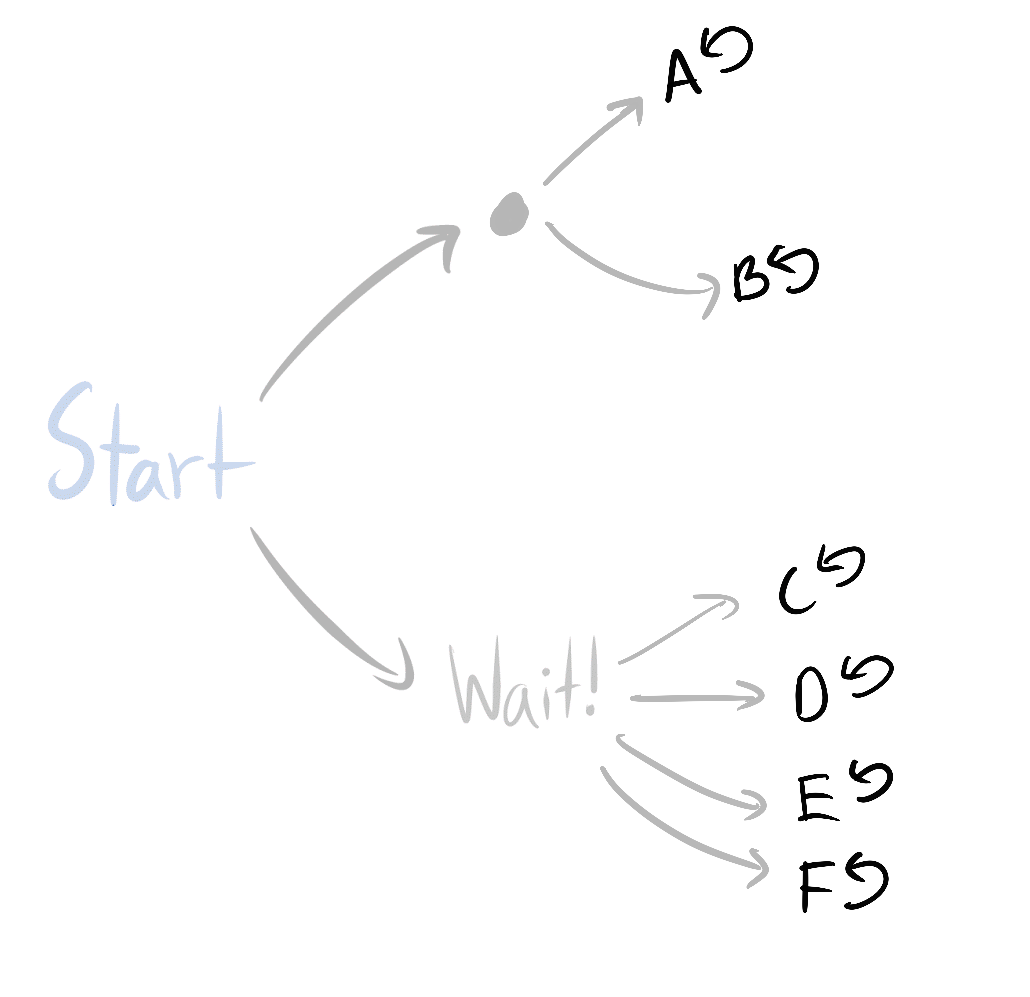For optimal policies, instrumental convergence is just as strong here.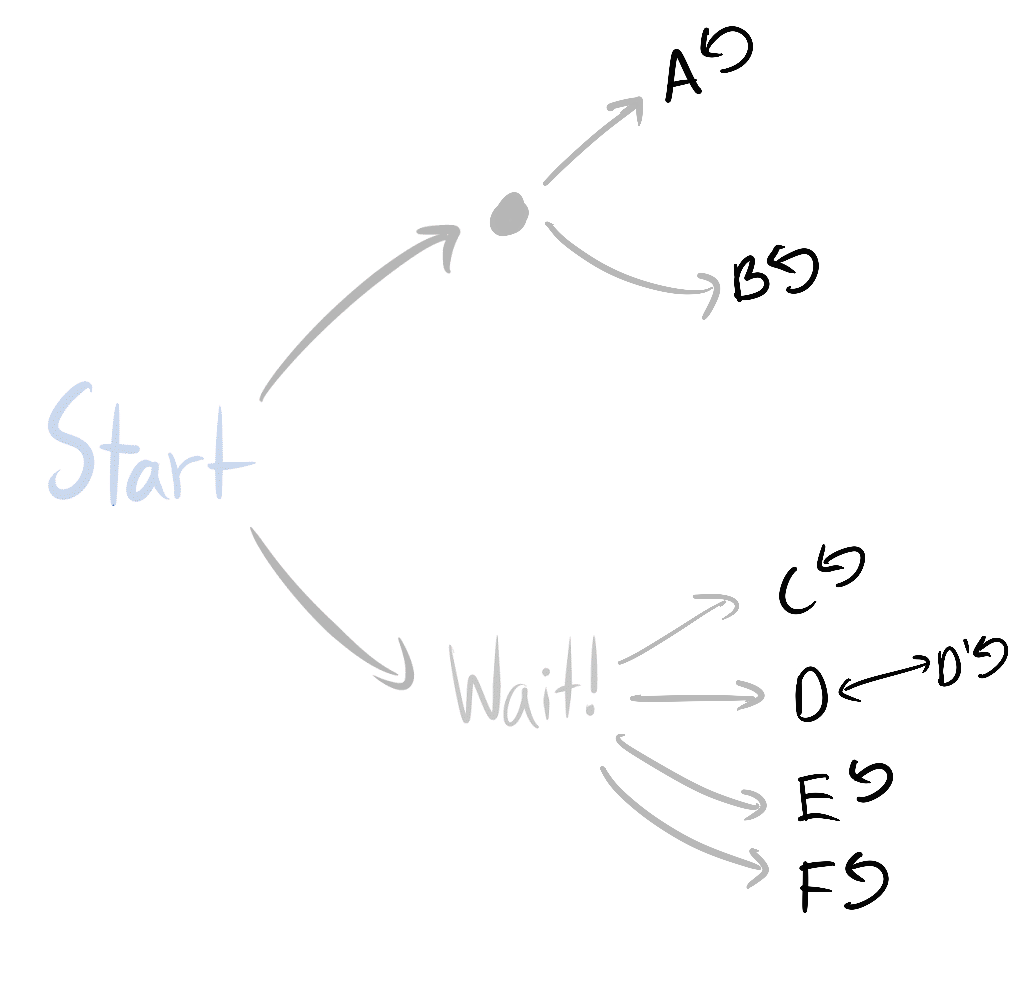And you can prove the same thing here as well - Wait! has at least twice as many ways of being average-optimal.

Similarly, you can do the inverse operations to simplify subgraphs in a way that respects the theorems.You could replace each of the circled subsets with anything you like, and the scaling law still holds (as long as the contents of each circle are replaced with the same new set of options).

This is the start of a theory on what state abstractions "respect" the theorems, although there's still a lot I don't understand there. (I've barely thought about it so far.)

# Note of caution, redux

Last time, in addition to the "how do combinatorics work?" question I posed, I wrote several qualifications:

• They assume the agent is following an optimal policy for a reward function
• I can relax this to -optimality, but  may be extremely small
• They assume the environment is finite and fully observable
• Not all environments have the right symmetries
• But most ones we think about seem to
• The results don't account for the ways in which we might practically express reward functions
• For example, often we use featurized reward functions. While most permutations of any featurized reward function will seek power in the considered situation, those permutations need not respect the featurization (and so may not even be practically expressible).
• When I say "most objectives seek power in this situation", that means in that situation - it doesn't mean that most objectives take the power-seeking move in most situations in that environment
• The combinatorics conjectures will help prove the latter

Let's take care of that last one. I was actually being too cautious, since the existing results already show us how to reason across multiple situations. The reason is simple: suppose we use my results to prove that when the agent maximizes average per-timestep reward, it's strictly optimal for at least 99.99% of objective variants to stay alive. This is because the death states are strictly suboptimal for these variants. For all of these variants, no matter the situation the agent finds itself in, it'll be optimal to try to avoid the strictly suboptimal death states.

This doesn't mean that these variants always incentivize moves which are formally POWER-seeking, but it does mean that we can sometimes prove what optimal policies tend to do across a range of situations.

So now we find ourselves with a slimmer list of qualifications:

1. They assume the agent is following an optimal policy for a reward function
• I can relax this to -optimality, but  may be extremely small
2. They assume the environment is finite and fully observable
3. Not all environments have the right symmetries
• But most ones we think about seem to
4. The results don't account for the ways in which we might practically express reward functions
• For example, state-action versus state-based reward functions (this particular case doesn't seem too bad, I was able to sketch out some nice results rather quickly, since you can convert state-action MDPs into state-based reward MDPs and then apply my results).

It turns out to be surprisingly easy to do away with (2). We'll get to that next time.

For (3), environments which "almost" have the right symmetries should also "almost" obey the theorems. To give a quick, non-legible sketch of my reasoning:

For the uniform distribution over reward functions on the unit hypercube (), optimality probability should be Lipschitz continuous on the available state visit distributions (in some appropriate sense). Then if the theorems are "almost" obeyed, instrumentally convergent actions still should have extremely high probability, and so most of the orbits still have to agree.

So I don't currently view (3) as a huge deal. I'll probably talk more about that another time.

This should bring us to interfacing with (1) ("how smart is the agent? How does it think, and what options will it tend to choose?" - this seems hard) and (4) ("for what kinds of reward specification procedures are there way more ways to incentivize power-seeking, than there are ways to not incentivize power-seeking?" - this seems more tractable).

# Conclusion

This scaling law deconfuses me about why it seems so hard to specify nontrivial real-world objectives which don't have incorrigible shutdown-avoidance incentives when maximized.

FN quotes: I'm using scare quotes regularly because there aren't short English explanations for the exact technical conditions. But this post is written so that the high-level takeaways should be right.

Thanks to Connor Leahy, Rohin Shah, Adam Shimi, and John Wentworth for feedback on this post.

# 29

New Comment

As I understand expanding candy into A and B but not expanding the other will make the ratios go differently.

In probablity one can have the assumtion of equiprobability, if you have no reason to think one is more likely than other then it might be reaosnable to assume they are equally likely.

If we knew what was important and what not we would be sure about the optimality. But since we think we don't know it or might be in error about it we are treating that the value could be hiding anywhere. It seems to work in a world where each node is pretty comparably likely to contain value. I guess it comes from the effect of the relevant utility functions being defined in the terms of states we know about.

As I understand expanding candy into A and B but not expanding the other will make the ratios go differently.

What do you mean?

If we knew what was important and what not we would be sure about the optimality. But since we think we don't know it or might be in error about it we are treating that the value could be hiding anywhere.

I'm not currently trying to make claims about what variants we'll actually be likely to specify, if that's what you mean. Just that in the reasonably broad set of situations covered by my theorems, the vast majority of variants of every objective function will make power-seeking optimal.